## Sunday, August 5, 2012

### Ballistic Entry from Low Mars Orbit

Scope and Purpose

This study attempts to define the conditions at end-of-hypersonics for future manned Mars lander designs, which will be in the several tons, to a hundred tons or so, range of entry masses. This study would also apply to large unmanned cargo landers as well. The Martian atmosphere is unique, in that it is too thick to ignore from an entry heating standpoint, but too thin to help very much at all, with respect to aerodynamic drag deceleration forces.

The scope of this study covers ballistic coefficient ratios from those of the successful unmanned lander probes (around 100 kg/sq.m), through values appropriate to prior manned capsule designs (300 kg/sq.m), to the much larger values thought to be associated with very large landing vehicles appropriate to manned landings (500 to 2000 kg/sq.m). These entry estimates are not computer simulations, but very simplified “back-of-the-envelope” models, appropriate only to exploratory calculations.

The mission of interest is entry from a circular low Mars orbit (LMO), not direct entry from an interplanetary transfer orbit (those would penetrate to even lower altitudes, all other factors being equal). This entry trajectory of interest would be associated with an exploration-type mission to Mars, one based from orbit, with multiple landings made in the one trip. In this context, LMO is defined to be 200 km altitude. Results would likely be very similar for other orbital altitudes, although that is not explored here.

The main objective here is a definition of altitude-at-end-of-hypersonics, for any given ballistic coefficient, through a range of ballistic coefficient values thought to be applicable.

Methods and Results

Reference 1 presents (among other things) a 1956-vintage “back-of-the-envelope” entry model based on the relationship between density scale height and deceleration. Reference 2 evaluates that model as basically sound, but flawed in its closed-form predictions of peak convective heating, at least those results posited for it in reference 1.

As it turns out, there is great value in that 1956 model if convective predictions are made versus slant range, and numerically integrated for peak values. The peak deceleration predictions of that 1956 model are just fine. The entire process is easily done with a modern spreadsheet, as in reference 2.

End-of-hypersonics is assumed to be Mach 3 velocity, based on the blunt shape of a capsule with a relatively-flat, spherical-segment heat shield, not a narrow conical re-entry body like a warhead. For narrow conical shapes, the end-of-hypersonics is closer to Mach 5. Below end-of-hypersonics, drag coefficients become strong functions of Mach number, instead of virtually constant hypersonically.

These manned landers are expected to have much larger ballistic coefficients than the probes sent so far. These would tend to penetrate far deeper into the Martian atmosphere before decelerating to “mere supersonic” speed. These altitudes are closer to 5 km than the 25-30 km of the Mars lander probes. Those lower values of final altitude tend to violate the density-scale height model’s region of applicability of 25-60 km altitude, as given in reference 1. Yet, these entry results should still be more-or-less representative, even though I did not revise the density-scale height model.

Ballistic Coefficients

Reference 3 contains an analysis of ballistic coefficient data for the successful Mars probe landers, the old manned space capsules of the 1960’s and 1970’s, and extrapolations to much higher entry masses thought to be appropriate for manned lander vehicles at Mars. There is a significant disparity among the probes and the old manned space capsules, as regards ballistic coefficient vs. vehicle entry mass. Ballistic coefficient is defined as

β, kg/sq.m = (entry mass, kg) / [(blockage area, sq.m)(hypersonic drag coefficient CD)]

where it should be noted that the reference area for CD should be the blockage area.

This β disparity is not resolvable, but can be “allowed-for” in the spread of ballistic coefficients explored herein. The posted update to reference 3 defines the scope that disparity. The relevant plot from reference 3 is reproduced here as Figure 1, which shows the wide range of ballistic coefficient predictions made by the extrapolation equations of Reference 3. Suffice it to say that for a 60 metric ton (at entry) lander, ballistic coefficient might range from about 500 kg/sq.m to about 2000 kg/sq.m, with the “best-guess” recommended prediction being 1174 kg/sq.m.Figure 1 – Extrapolations of Beta-Equations to 300 Ton Entry Masses

Those “best-guess” recommendations from reference 3, as updated, would indicate that a 60 ton lander might be a 7.1 m diameter conical shape about 7 m tall. It might have a ballistic coefficient of something like 1174 kg/sq.m (as predicted by the very best guess from Reference 3) at a hypersonic drag coefficient CD of 1.3 (similar to Apollo). Overall, it might resemble the conical Apollo capsule in form. Such a shape would have enhanced stability landing on rough ground, due to the low center of gravity location.

The heat shield radius of curvature would be substantially larger than 10 m, although a “nose radius” of 1 m (used only in the heating estimate) was retained in the entry model. It is the trajectory dynamics, not the convective heating estimates, that are of interest here. The heating trend will have the right shape, but the wrong values, without this correction.

Entry “Transfer” Orbit

The entry trajectory considered here is illustrated in Figure 2. A very small retro velocity change converts a 200 km circular orbit to a transfer ellipse that intersects the surface of Mars. Using the entry interface altitude of reference 1, and the relationship among orbital parameters given in the figure, the location of entry interface can be defined, which leads to the trajectory depression angle below horizontal, at interface.Figure 2 – Entry Trajectory and Parameters

The velocity at interface is also easily estimated. Values are Vatm = 3.469 km/s, hatm = 135 km, and γ = 1.63 degrees. Those are the starting conditions for the entry model. The back-of-the-envelope entry model of Reference 1 then uses a straight-line trajectory representation in 2-D Cartesian coordinates for its calculations.

The Martian atmosphere is highly variable. I used the “typical” values given in reference 1, augmented by computations of speed-of-sound vs altitude. These data were posted in reference 4. For altitudes from 10 to 60 km, speed of sound in this Mars atmosphere model is very linear, and its extrapolation to the surface is pretty close. That linear model was used to compute Mach number in the lower range of altitudes in the entry model:

c, m/s = -0.74(h, km) + 236.4

Salient Results

Not surprisingly, altitude for end-of-hypersonics is closer to 25-30 km for the lander probes which cluster around ballistic coefficient values near 100. This matches what really happened very well. This model predicts 29 km for β = 100 kg/sq.m. The historic space capsules Mercury, Gemini, and Apollo cluster around β = 300, for which the model predicts about 19 km. The β = 500-2000 kg/sq.m range encompassing manned Mars landers predicts altitudes from about 15 km all the way down to about 3 km. This curve is shown in Figure 3. It is easy to see that end-of-hypersonics occurs at surface impact, given a sufficiently-large ballistic coefficient (somewhere above about 2500-3000 kg/sq.m).Figure 3 – Variation of End-of-Hypersonics Altitude with Ballistic Coefficient

The entry model was run for β = 100, 300, 500, 1000, 1500, and 2000 kg/sq.m. Plots were made of the spreadsheet image and the data plots, at each β. These are given below at the end of this article.

Beta 100 Study

Beta values near 100 correspond to those of the various lander probes (63 to 115). The terminal altitude is 29 km. These were included to show that the analysis produces realistic results. These are end-of-hypersonics altitudes near 25-30 km. The large MSL “Curiosity” lander is known to push the state-of-the-art for effective parachute deceleration of any kind on Mars, at about β = 115. These are ring-sail parachutes designed for opening at supersonic speeds, whose terminal velocity is still supersonic on Mars.

Five figures are presented: one a collage of spreadsheet images containing the numerical results of the entry model, the other four being plots from that spreadsheet. These are Figure 4 (spreadsheet), Figure 5 (range and slant range vs altitude), Figure 6 (velocity vs slant range), Figure 7 (deceleration gees vs slant range), and Figure 8 (heating data vs slant range).

Beta 300 Study

This roughly corresponds to the historic capsules Mercury, Gemini, and Apollo (246 to 374), if such configurations were to be used on Mars. (Most likely, they will not be.) The terminal altitude is 19 km. Five figures are presented: one a collage of spreadsheet images containing the numerical results of the entry model, the other four being plots from that spreadsheet. These are Figure 9 (spreadsheet), Figure 10 (range and slant range vs altitude), Figure 11 (velocity vs slant range), Figure 12 (deceleration gees vs slant range), and Figure 13 (heating data vs slant range).

Beta 500 Study

This corresponds roughly to the new Spacex Dragon capsule rigged as a one-way lander on Mars, using the Super Draco thrusters as braking rockets. (I get about 453 kg/sq.m for a 3.6 m diameter, a guess for CD = 1.3 similar to Apollo, and loaded to about 6 tons at entry. It’s about 380 kg/sq.m if you guess a CD nearer the 1.55, similar to Mercury and Gemini with the taller shapes.) It is not very credible as the ballistic coefficient of a much larger 60 ton lander. The terminal altitude is 15 km.

Five figures are presented: one a collage of spreadsheet images containing the numerical results of the entry model, the other four being plots from that spreadsheet. These are Figure 14 (spreadsheet), Figure 15 (range and slant range vs altitude), Figure 16 (velocity vs slant range), Figure 17 (deceleration gees vs slant range), and Figure 18 (heating data vs slant range).

Beta 1000 Study

This is a little bit lower ballistic coefficient than what might be credible for a short, squat 60 ton lander. The terminal altitude is 9 km. Five figures are presented: one a collage of spreadsheet images containing the numerical results of the entry model, the other four being plots from that spreadsheet. These are Figure 19 (spreadsheet), Figure 20 (range and slant range vs altitude), Figure 21 (velocity vs slant range), Figure 22 (deceleration gees vs slant range), and Figure 23 (heating data vs slant range).

Beta 1500 Study

This is a little bit higher ballistic coefficient than what might be expected for a short, squat 60 ton lander. The terminal altitude is 6 km. Five figures are presented: one a collage of spreadsheet images containing the numerical results of the entry model, the other four being plots from that spreadsheet. These are Figure 24 (spreadsheet), Figure 25 (range and slant range vs altitude), Figure 26 (velocity vs slant range), Figure 27 (deceleration gees vs slant range), and Figure 28 (heating data vs slant range).

Beta 2000 Study

This is at the upper end of what might be credible for a short, squat 60 ton lander. It would be fairly representative of a taller design, one that we have no landing experience with. The terminal altitude is 3 km. Five figures are presented: one a collage of spreadsheet images containing the numerical results of the entry model, the other four being plots from that spreadsheet. These are Figure 29 (spreadsheet), Figure 30 (range and slant range vs altitude), Figure 31 (velocity vs slant range), Figure 32 (deceleration gees vs slant range), and Figure 33 (heating data vs slant range).

References

1. “Atmospheric Environments for Entry, Descent, and Landing (EDL)”, C. G. Justus (NASA Marshall) and R. D. Braun (Georgia Tech), June, 2007.
2. G. W. Johnson, “Back of the Envelope Entry Model”, article dated 7-14-12, posted to http://exrocketman.blogspot.com.
3. G.W. Johnson, “Rough Correlation of Entry Ballistic Coefficient vs. Size for “Typical” Mars Landers”, article dated 7-25-12, posted to http://exrocketman.blogsapot.com.
4. G. W. Johnson, “Atmosphere Models for Earth, Mars, and Titan”, article dated 6-30-12, posted to http://exrocketman.blogspot.com.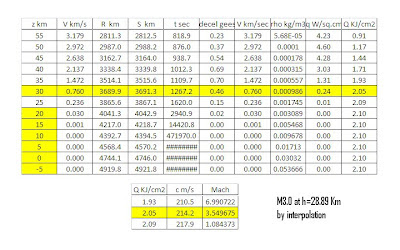Figure 4 – Spreadsheet Image, Beta 100Figure 5 – Range and Slant Range vs Altitude, Beta 100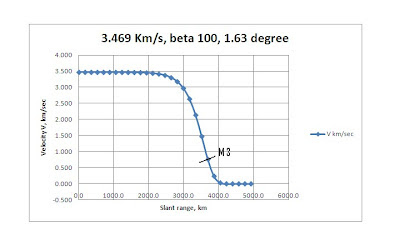Figure 6 – Velocity vs Slant Range, Beta 100Figure 7 – Gees vs Slant Range, Beta 100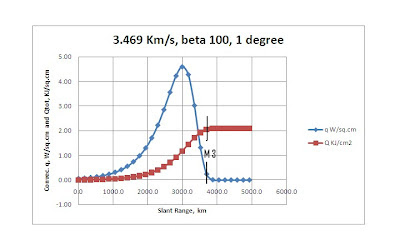Figure 8 – Heating vs Slant Range, Beta 100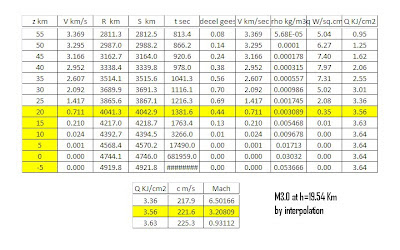Figure 9 – Spreadsheet Image, Beta 300Figure 10 – Range and Slant Range, Beta 300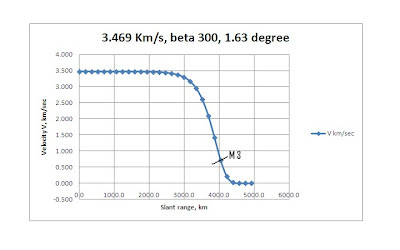Figure 11 – Velocity vs Slant Range, Beta 300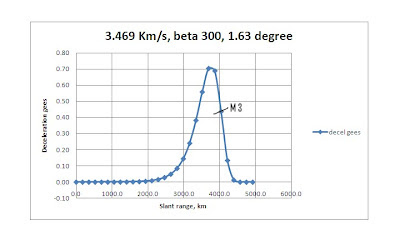Figure 12 – Gees vs Slant Range, Beta 300Figure 13 – Heating vs Slant Range, Beta 300Figure 14 – Spreadsheet Image, Beta 500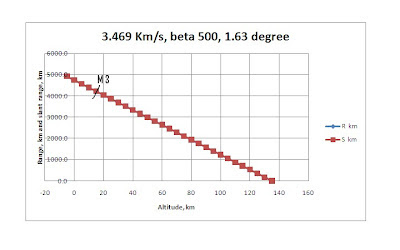Figure 15 – Range and Slant Range vs Altitude, Beta 500Figure 16 – Velocity vs Slant Range, Beta 500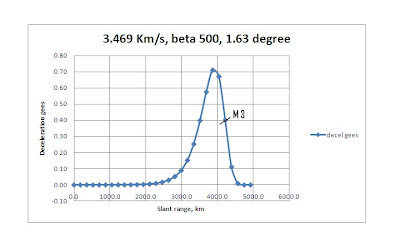Figure 17 – Gees vs Slant Range, Beta 500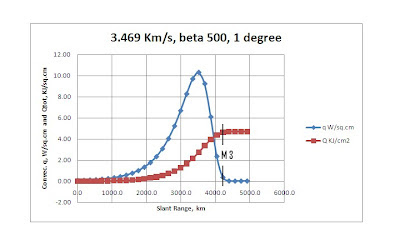Figure 18 – Heating vs Slant Range, Beta 500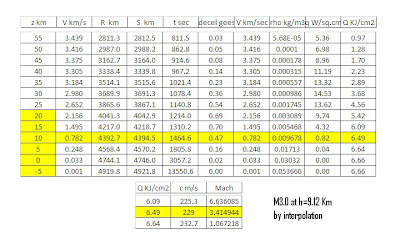Figure 19 – Spreadsheet Image, Beta 1000Figure 20 – Range and Slant Range vs Altitude, Beta 1000Figure 21 – Velocity vs Slant Range, Beta 1000Figure 22 – Gees vs Slant Range, Beta 1000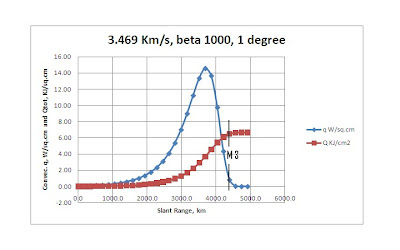Figure 23 – Heating vs Slant Range, Beta 1000Figure 24 – Spreadsheet Image, Beta 1500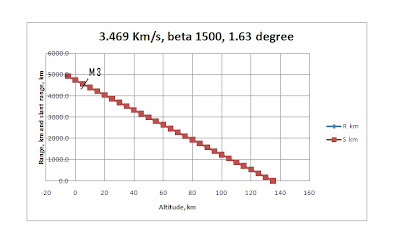Figure 25 – Range and Slant Range vs Altitude, Beta 1500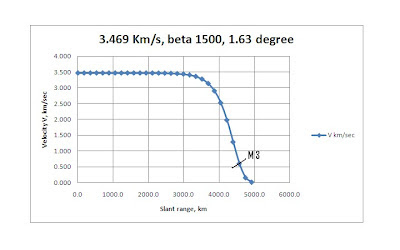Figure 26 – Velocity vs Slant Range, Beta 1500Figure 27 – Gees vs Slant Range, Beta 1500Figure 28 – Heating vs Slant Range, Beta 1500Figure 29 – Spreadsheet Image, Beta 2000Figure 30 – Range and Slant Range vs Altitude, Beta 2000Figure 31 – Velocity vs Slant Range, Beta 2000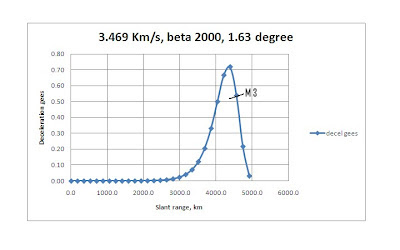Figure 32 – Gees vs Slant Range, Beta 2000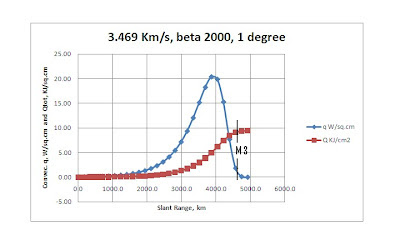Figure 33 – Heating vs Slant Range, Beta 2000